##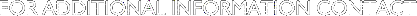### DARREN GARBER

ALL INFORMATION IS COPYRIGHTED GARBER ASTRONAUTICS 1998-2002

REPRODUCTION IN ANY MEDIUM WITHOUT PERMISSION IS STRICTLY FORBIDDEN

# A

a - the semi-major axis

angular momentum vector - h - the resulting cross product of the postion and velocity vectors which is a constant. h is normal to the orbit plane. The angle between the primary body's polar axis and h is the inclination.

apogee - the farthest point on an orbit from the earth

apoapsis - the farthest point on an orbit from the primary body. For earth orbits apoapsis is called apogee.

apparent solar day - solar day

argument of perigee -, -a COE, the angle from the longitude of the ascending node to perigee for earth orbits

argument of periapsis -, - the generic term for the COE that is defined as the angle from the ascending node to the closest point the orbit makes with the primary body, for earth orbitsthis term is called the argument of perigee

ascending node - the point on the orbit where the spacecraft crosses the equatorial plane moving from the southern hemisphere to the northern hemisphere. 180 degrees from the ascending node is the descending node.

# B

ballistic coefficient - a measure of how aerodynamic a satellite is for computing drag effects and perturbations.

# D

descending node - the point in the orbit where the spacecraft crosses the equatorial plane moving from the northern hemisphere to the southern hemisphere. 180 degrees from the descending node is the ascending node.

DU - D istance U nits - the mean equatorial radius of the primary body, for earth orbits one DU is equal to one earth radii.

# E

e -eccentricity

ECI - Earth Center Inertial

ECEF - Earth Centered Earth Fixed

Earth Centered Inertial - ECI - a coordinate system in which the primary or I axis is directed towards the First Point of Aries and the rotational rate of the earth is NOT taken into account.

Earth Centered Earth Fixed - ECEF - a coordinate system in which the primary or I axis is tied to the Prime Meridian and the rotational rate of the earth IS taken into account.

earth radii - ER - the mean distance from the center of the earth to the surface - 6378.137 kilometers or 1

eccentric anomaly - the angle between two points on an ellipse measured from a focus.

eccentricity - a measure of how circular (or elliptical) an orbit is

ecliptic - the orbital plane of the earth about the sun. The ecliptic does not lie in the same plane as the equator. The equatorial plane is inclined to the ecliptic by 23.5 degrees - the earth's axial tilt. The orbits of the planets and moon are within a few degrees of the ecliptic plane.

ellipse - the conic section defined by passing a plane through a right circular cylinder at angle between 0 and 90 degrees. Most orbits to a first order approximation are ellipses.

equatorial plane - the projection of the earth's equator out into space. The equatorial plane is inclined 23.5 degrees to the ecliptic.

# F

First Point of Aries -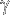- arbitrary direction in which the inertial I axis points for the Solar System.2000 years ago on the vernal equinox the sun rose in the constellation Aries. The zodical sign for Aries isand for historical reasons the symbol of the Ram continues to be used to define the principle axis in celestial coordinate systems.

flight path angle -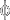- the angle between the velocity vector and the local horizon. In aeronautics this is called the angle of attack.

# G

ground track - the line traced out by a spacecraft's orbit against the earth's surface.

# H

HEO - Highly Eliptical Orbit

# I

i - inclination

inclination - the angle between the orbit plane and the equator of the primary body, for earth orbits the inclination is equal to the highest lattitude that the spacecraft's ground track crosses.

# J

Julian Date - the basis of time for astrodynamics. The number of days since 12:00 on 1 January 4713 B.C. The Julian Day for 1 January 2000 at noon is 2451545.0. Julian Days are measured from noon to noon so that astronomers can have all of their observations during the same day.

# K

Kepler's Laws of Planetary Motion - named for Johannes Kepler who determined the following three laws of two body motion:

• First Law - the orbit of each planet is an ellipse with the sun at a focus
• Second Law - the line joining the planet to the sun sweeps out equal areas in equal times
• Third Law - the square of the a planet's period is proportional to the cube of its mean distance from the sun.

# L

LEO - Low Earth Orbit

longitude of the ascending node -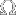, - the angle between the Greenwich Meridian and the ascending node. LAN realtes the orbits orientation to the Prime Meridian. RAAN relates the orbits orientation relative to the stars.

# M

MEO - Medium Earth Orbit

mean anomaly - an expression containing the eccentric anomaly, E, and used to determine time of flight calculations based on Kepler's Second Law. It is also the angle between perigee and a point on a circle circumscribing the ellipse (orbit). Mean anomaly is equal to E andat periapsis (all equal 0 degrees) and

at apoapsis (all equal 180 degrees).

Molniya - HEO

# N

nadir - the point directly below in the direction of the center of the earth. For spacecraft the arbitrarily defined 'down' direction. This is usually defined as the direction pointing toward the center of the primary body. Opposite of nadir is zenith.

# O

orbit - the path traced out by a body moving about a primary body. Keplerian orbits follow conic sections.

orbit plane - the plane defined by the motion of an object about a primary body. The position and velocity vectors lie withing the orbital plane while the angular momentum vector is a right angles to the orbital plane.

# P

penumbra - the edge of the primary body's shadow.

periapsis - the closest point in an orbit to the primary body. For earth orbits periapsis is known as perigee.

perigee - the closest point on an orbit to the earth.

period - the time required for one body to orbit the primary body. The period is related to the semi-major axis as defined by Kepler's Third Law.

primary body - the object which is the gravitational center of the system. The sun is the primary body for the Solar System and the earth is the primary body for almost all satellite operaitons.

Prime Meridian - the line of longitude that runs through Greenwich, England and from which longitude is

measured east or west. 180 degrees from the Prime Meridian lies the International Date Line.

# Q

quaternions - a mathematics that allows for the rotation of vectors in three dimensions without the problems of singularities associated with Euler Angles.

# R

RAAN - Right Ascension of the Ascending Node

radius - the distance from the center of a circle or sphere to its edge. The distance from the center of the earth to the surface is 1 DU.

Right Ascension of the Ascending Node - the angle between the ascending node and the First Point of Aries. RAAN relates the orbits orientation relative to the stars. LAN realtes the orbits orientation to the Prime Meridian.

rotational rate - the time it takes for a body to rotate once about a particular axis. The rotational rate for the earth is 1 solar day.

# S

selen - a prefix meaning "of or pertaining to the Moon"

semi-major axis - one half the distance across the longest line through an ellipse, for circular orbits the semi-major axis is the radius of the circle, the semimajor axis is usually measured in km or DU

solar day (apparent) - the time between two successive overhead transits of the sun for a given line of longitude. A solar day is shorter than a sidereal day.

# T

TOD - True of Date

true anomaly -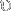, - the angular distance from periapsis to the actual position of the satellite in its orbit.is equal to E and M at periapsis (all equal 0 degrees) and at apoapsis (all equal 180 degrees).

True of Date - TOD - the most accurate coordinate system used to define a body's position relative to the center of the Earth. This coordinate system incorporates the earth's rotation, UTC corrections, precession, nutation, and polar wander.

two body problem - the simplest orbit which includes the primary body and the body in question. NO other forces are included in the calculation. Kepler's Laws are based on solving for the two body problem. These solutions are conic sections.

# U

umbra - the shadow of the primary body.

# V

vernal equinox - the first day of spring. The day in which the sun appears to move from the southern hemisphere to the northern hemisphere. The apparent ascending node of the sun as viewed from earth. This occurs around 21 March. On this day the earth is aligned with.

# W

World Geodetic Survey - the survey conducted by the Defense Mapping Agency in 1984 that includes a standardized set of constants and a potential model for the earth to degree and order 41.

# Z

zenith - the point directly overhead. For spacecraft this arbitrarily defined as 'up' and away from the primary body. The opposite of zenith is nadir.